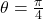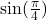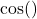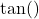# Function Pointers and Member Function Pointers

Material on function pointers and member function pointers is available in a variety of books and online sources. We're doing a review here because it makes understanding boost::function and boost::bind much easier.

## Function Pointers

Given:

[cpp]
double sin(double theta);
double cos(double theta);
double tan(double theta);
[/cpp]

We wish to declare a that can refer to any of those functions. As a practical matter, function declarations include typedef. The code below creates a function pointer that takes a double as an argument and returns a double.

1. First, we declare a function pointer using a typedef.
2. Second, we assign a variable of type TrigFunc to the address of sin().
3. Create an angle,in radians.
4. Use the function pointer to calculate5. Create an array of function pointers to each of,,.
6. Invoke each of the trigonometric functions by dereferencing elements of the TrigFunc array.

[cpp]
#includevoid functionPointerExample() {
typedef double (*TrigFunc)(double);
TrigFunc pS = &sin; // notice that there is no * on pS
double theta = 3.1415/4;
double h = (*pS)(theta); // we have to dereference pS with *

static const int N=3;
TrigFunc func[N] = { &sin, &cos, &tan };
double result[N];
for (int i=0; i

## Member Function Pointers

What is a class? A class is data coupled with the functions that operate on that data. With this in mind, consider that C++ requires an instance pointer to use (non-static) pointers to non-static member functions. Because of the possibility of virtual member functions and the vtable (or its moral equivalent), there is no guarantee on the size of member function pointers. I've seen them range from 8bit to 128bit on different platforms. Do not assume that a fits in an int.

[cpp]
void memberFunctionPointerExample() {
using namespace std;
// member function pointer not only has a signature but
// also a class. An instance of the class is required to
// call a non-static member function pointer.
typedef const char* (string::*MemberFunc)() const ;
// Address is of the function const char* std::string::c_str() const;

// Make a class instance
string helloStr("hello");
// Using the string instance to provide context,
// invoke the member function pointer.
const char* pChar = (helloStr.*pMF)();
} // end memberFunctionPointerExample()
[/cpp]